# Artificial Intelligence Applications in O&M of PV Plants

In the solar energy sector, AI is now being used to help push solar PV toward grid parity in markets throughout the world through two complementary approaches.

# Artificial Intelligence Applications in O&M of PV Plants

In the solar energy sector, AI is now being used to help push solar PV toward grid parity in markets throughout the world through two complementary approaches.

# Luis del Aguila

June 9, 2020

Artificial intelligence (AI) is already present in our daily life, from voice-assisted applications to the automation of trading decisions in the financial sector. In one study (1) published in 2017, PWC company estimated that AI could contribute up to \$15.7 trillion to the global economy by 2030, which only shows the high potential of this technology that leads the transformation of the industry 4.0.

In the global solar energy sector, AI is now used to push solar PV towards grid parity in markets through two complementary approaches.

One is energy production and demand forecasts: The reduction of uncertainty in power production and grid power demand forecasts enable smarter operations (2) by reducing unexpected curtailment and maximizing the penetration of renewables.

The second is the optimization of the PV plant performance: This is possible by identifying failures of the overall PV system (inverters, DC subsystems, and more). Not only this approach does intend to increase PV plant production, but it also aims to decrease the O&M costs by providing early failure detection. Besides, it detects components with the lowest performance, which need to be repaired or replaced.

In the literature, there are three main groups of modern methodologies for monitoring and diagnosis of PV plants (3): (a) electrical methods based on the direct measurement of electrical parameters; (b) AI methods; (c) thermal analysis, mostly concerning thermal images captured via air drones.

In the electrical methods, the algorithms that are used to model, control and predict the energy system's performance often involve complicated differential equations. This process demands immense computer power and extended time requirements (4). However, a different approach is to employ AI techniques that can “learn” the key information patterns and relationships in a multidimensional information domain, such as artificial neural networks, genetic algorithms or random forests.

Several studies have proved various applications of AI in the solar energy sector, thanks to the diversity and flexibility of Machine Learning (ML) algorithms. Some of these applications are the detection of incidences, either using unsupervised learning (5) or comparing the measured data with simulated electrical measurements (6). Other examples include short-circuiting fault detection of PV-arrays by using artificial neural networks (ANN) (7); hierarchical context-aware anomaly diagnosis methods to identify operating states of individual strings (8) automatically; or prediction of soiling effects with a Bayesian neural network and polynomial regressions (9).

Finally, the third approach of thermal analysis could also be optimized using Convolutional Neural Networks (CNN), which is proven to be highly efficient in classifying thermal images in medical applications (10). It could also be adapted to detect and classify incidences from thermographic images, following a scheme like the one shown below.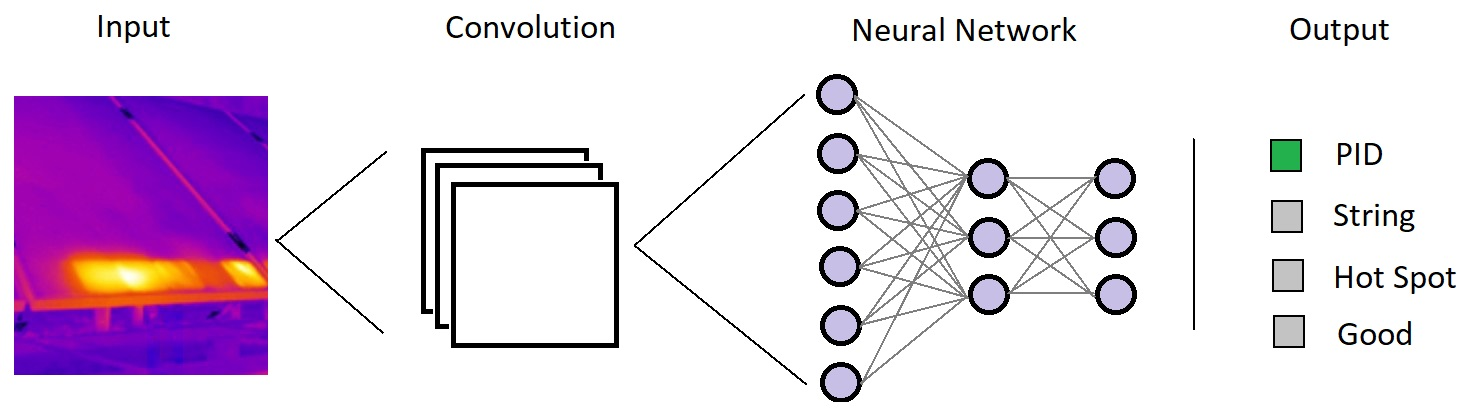Fig 3. High-level scheme of the application of CNN to classify thermographic images from PV modules

The next section shows a basic example on how to train a model out of some meteorological data from a small PV plant to predict the power generation of a PV-array and how we can optimize the model and use it to detect energy losses.

### Power Generation Model

One must know how the ML algorithm works to choose the type of algorithm that suits their model the best. For instance, a decision tree model forms a prediction based on multiple yes/no questions that depend on certain hyperparameters, while a linear model assumes a linear relationship amongst variables. Additionally, it is essential to identify the intrinsic nature of the system; in this case, the physical principles that link the irradiance and temperature to the power generation in a PV array. It is vital due to the following reasons:

1.    The interpretation of the model often helps determine what the root causes of the power losses are, and what variables are more influenced when there is a strong deviation between predicted and measured data.

2.   The model should generalize well in case of similar conditions. For instance, in this study, we could include the windspeed to train the model, as an additional source of information. However, it is not desirable for the model to give so much importance to this variable when making a prediction. It could happen if, for some reason, it is correlated to some other relevant variable (like the module temperature) - especially if we have very few data.

In this study, we use the 1-diode model equations to model the behavior of the PV array, with the following relationships: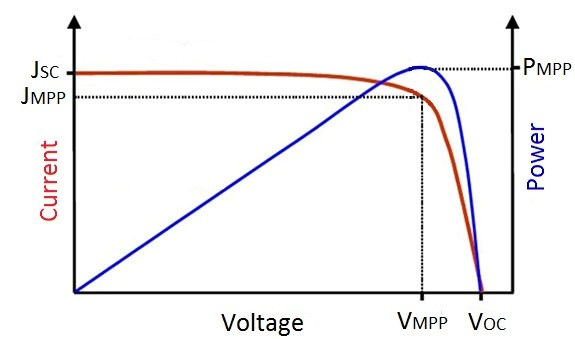Fig 4. Graphic representation of the short circuit current, open circuit voltage and maximum power points in a solar cell JV curve

Here, I0 represents the saturation current of the modules, and IL is the photogenerated current, which is proportional to the irradiance. Thus, under normal operating conditions, we can approximate the generated power to be proportional to the irradiance (Rad) and the module temperature (Tmod), which are the relevant variables in our dataset.

This relationship is represented in the next figure, where the DCPower has been plotted against the irradiance, and the color is set according to the module temperature. It is noticeable that, although the DCPower mainly depends on the irradiance, the higher the module temperature, the lower the DCPower is, especially at high irradiances.

The following step is to train the model and optimize it by adding/subtracting some variables; to see what effect they have on the results, analyze their limitations and interpret the results. The next figures represent the power generation in a sunny day (left), the Power-Irradiance curve (right), and the predictions according to three different models: two linear model and an XGBoost (11).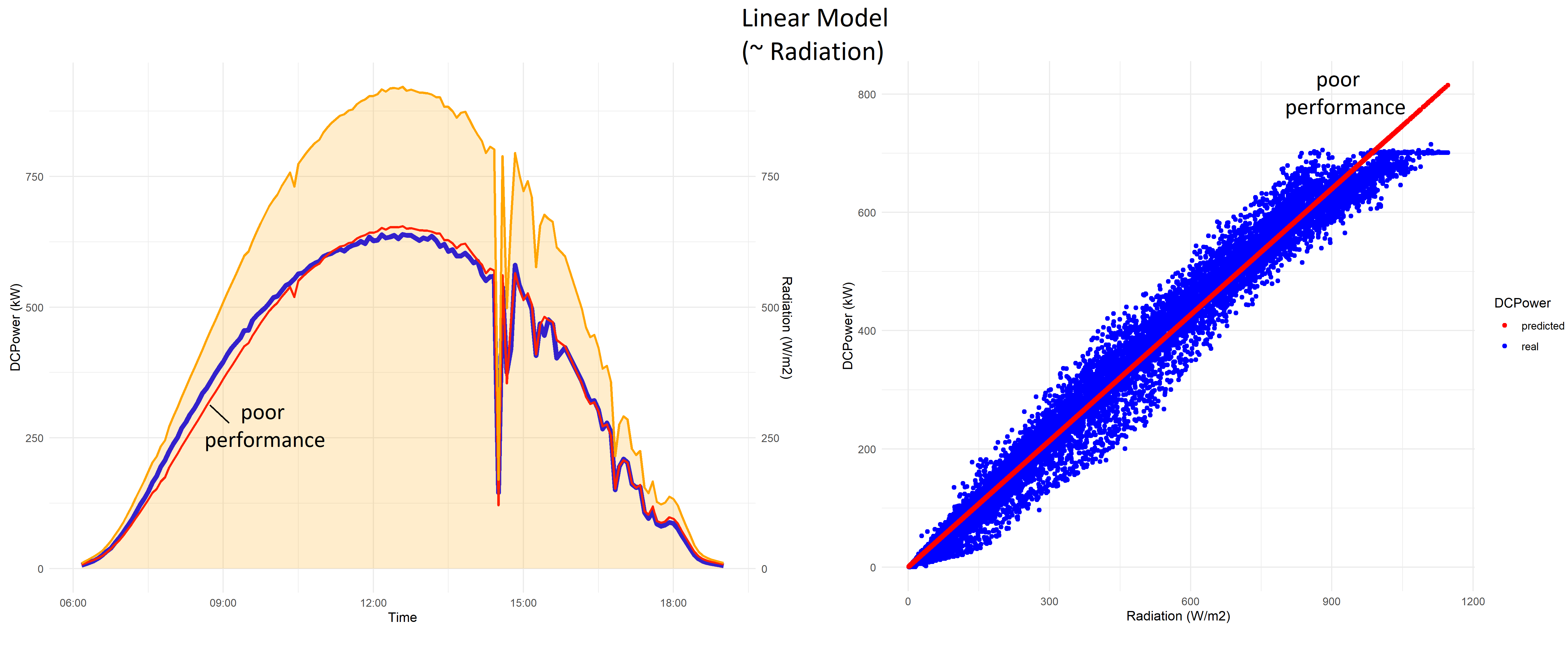Fig 6. DCPower predicted with a linear model (~Radiation) (red) compared to measured DCPower (blue) in a power generation curve in a high irradiance (orange) day (left), and DCPower-Irradiance relationship for several months (right)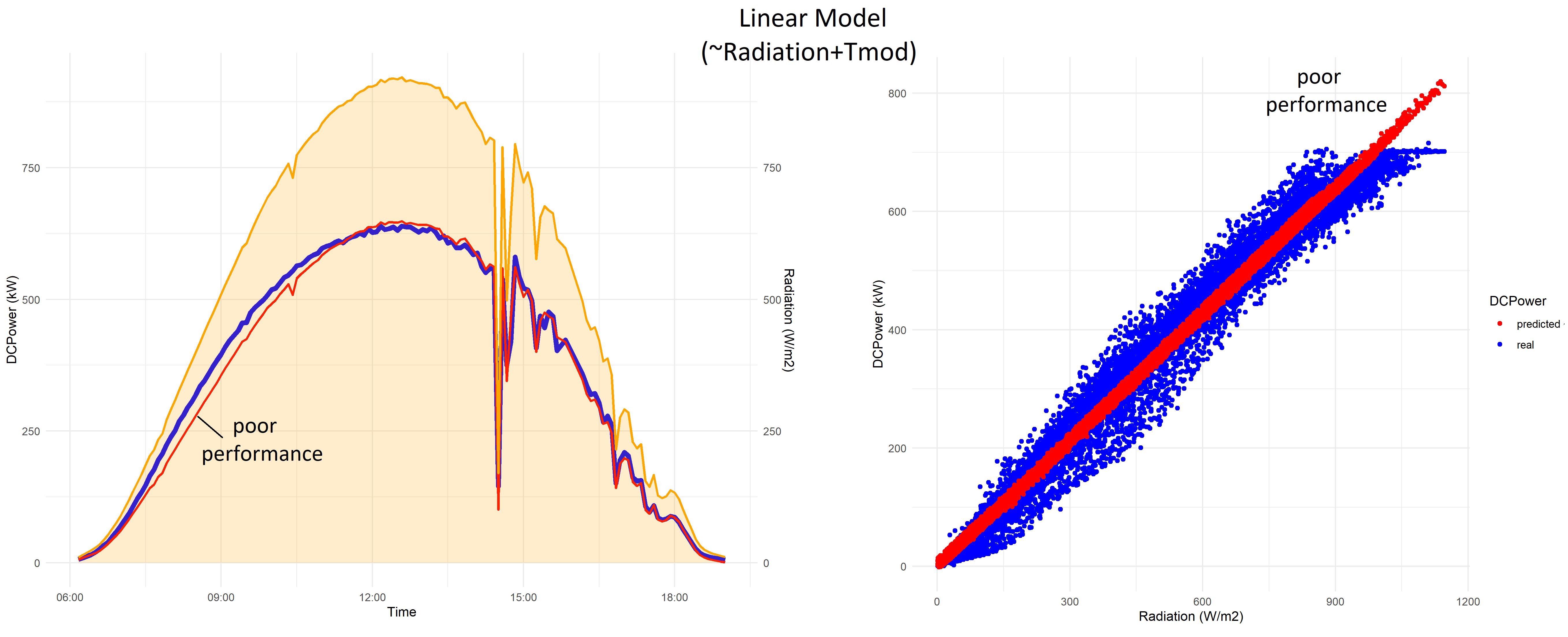Fig 7. DCPower predicted with a linear model (~Radiation + Tmod) (red) compared to measured DCPower (blue) in a power generation curve in a high irradiance (orange) day (left), and DCPower-Irradiance relationship for several months (right)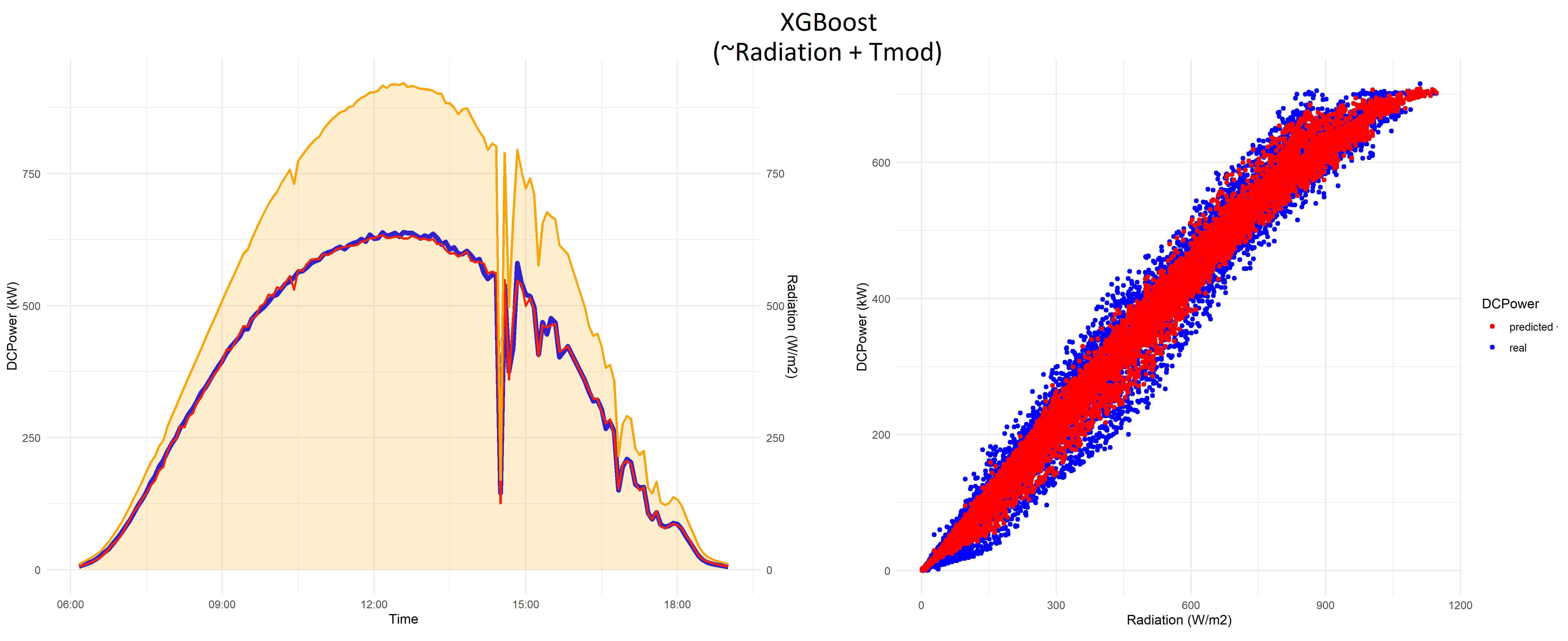Fig 8. DCPower predicted with aXGBoost model (~Radiation + Tmod) (red) compared to measured DCPower (blue) in apower generation curve in a high irradiance (orange) day (left), andDCPower-Irradiance relationship for several months (right)

It must be noted that the data used to train these models were processed to eliminate inconsistencies, outliers or any incidences that are present in the PV plant since otherwise, we would be training our model with fake ideal operation points.

Although the first linear model does not do a bad job at predicting the DCPower, the module temperature included in the second one does not seem to help much, especially in the mornings. This difference might be caused by other components, such as the wires or the inverter which controls the Maximum PowerPoint (MPP) of the IV curve, and so, influences the power generation.

However, thanks to the wide variety of ML models, one can also optimize the models that best adapt to the problem. In this case, the third model is an XGBoost, a gradient boosting machine algorithm, which even seems to be able to learn the power limitation at high irradiance due to the maximum nominal power of the inverter.

The next table summarizes the Root Mean Squared Errors (RMSE) of each model, which is one of the most common metrics to measure the performance of a regression model.

Now, we can use the model to predict the power generation and compare it with real data, seeking for energy losses and characterizing them by answering questions like:

1.    Do the differences slowly increase with time or appear suddenly? It could be the case of a sudden string disconnection, or a long-term module degradation.

2.    Do the differences influence only the current or the voltage, too? For example, while soiling reduces only the amount of light that reaches the solar cells, decreasing the photogenerated current, PID can also have a strong impact on the voltage.

3.    Are the energy losses constant throughout the day, or only prominent during morning/afternoon? Are they related to the module temperature?

4.    Do these differences appear in other elements of the PV plant? For example, the inverter clipping could limit the power generation of several strings.

The next figure shows a few examples of power losses: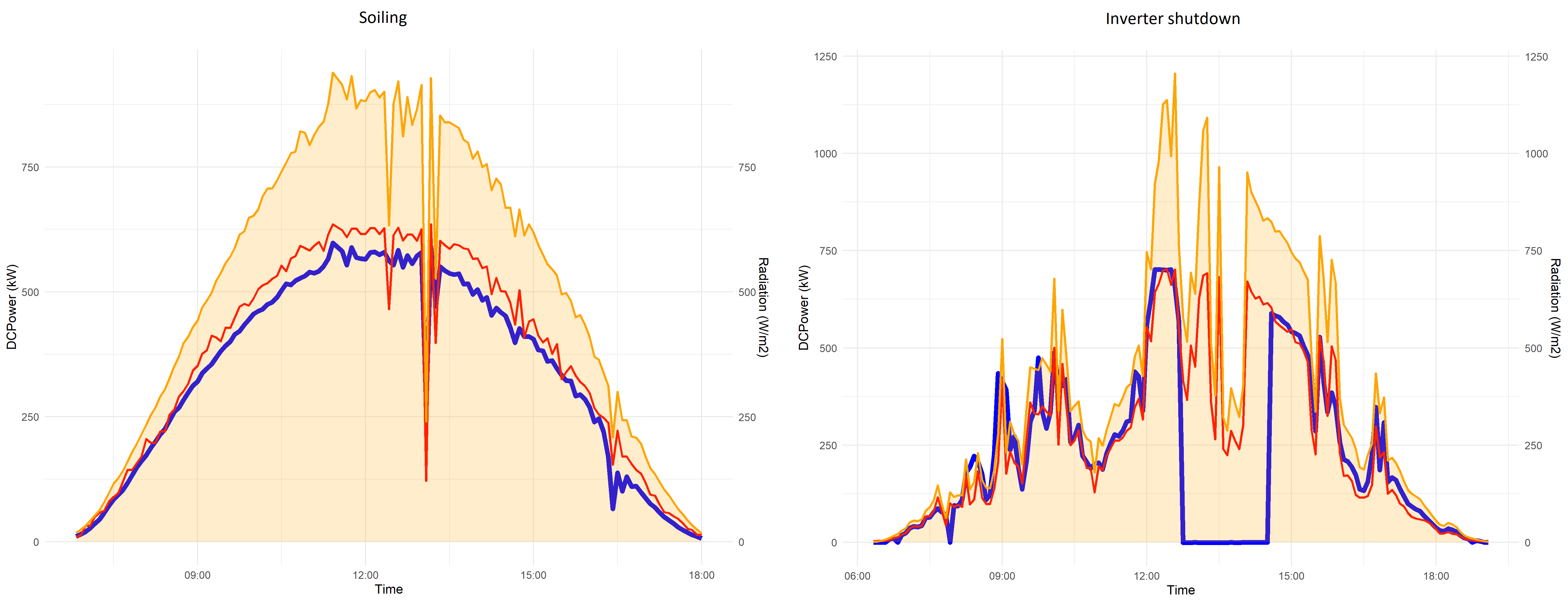Fig 9. Examples of differences between measured and predicted DCPower curve due to soiling (left), and inverter shutdown (right)

### Conclusions

The example presented here intends to show a basic approach on how to use ML techniques to detect energy losses in PV installation by analyzing the nature of the problem, training a model out of meteorological data and detecting its limitations when predicting data.

However, in real-life applications, the use of ML models requires other steps that are omitted here for simplicity. These are such steps as the data quality analysis (data cleansing, imputation of missing or constant values, outliers); feature engineering (principal component analysis, linear discriminant analysis, and more); the model selection, optimization, and validation. And even the combination of several algorithms to guarantee accurate and reliable results for detecting incidences in PV plants.

Cover photography by Christopher Burns.